Courses

# Quant Mock Test - 6

## 15 Questions MCQ Test Mock Test Series for CLAT 2020 | Quant Mock Test - 6

Description
This mock test of Quant Mock Test - 6 for CLAT helps you for every CLAT entrance exam. This contains 15 Multiple Choice Questions for CLAT Quant Mock Test - 6 (mcq) to study with solutions a complete question bank. The solved questions answers in this Quant Mock Test - 6 quiz give you a good mix of easy questions and tough questions. CLAT students definitely take this Quant Mock Test - 6 exercise for a better result in the exam. You can find other Quant Mock Test - 6 extra questions, long questions & short questions for CLAT on EduRev as well by searching above.
QUESTION: 1

### Directions: Study the table to answer the given questions: Number of cars sold (in thousand) by 5 showrooms during 5 months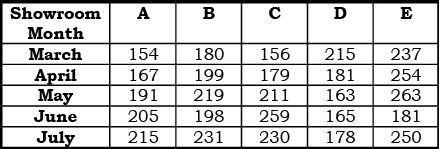Q. What is the average number of cars sold (in thousand) by showroom C in all the given months together?

Solution: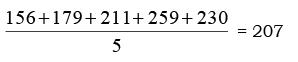QUESTION: 2

### Directions: Study the table to answer the given questions: Number of cars sold (in thousand) by 5 showrooms during 5 monthsQ. By what percent the number of cars sold by showroom B increases from March to July?

Solution: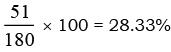QUESTION: 3

### Directions: Study the table to answer the given questions: Number of cars sold (in thousand) by 5 showrooms during 5 monthsQ. The number of cars sold by showroom E increased by 8% from July to August and by 20% from August to September. How many cars were sold (in thousand) by store E in September?

Solution:

August = 108% of 250 = 270
September = 120% of 270 = 324

QUESTION: 4

Directions: Study the table to answer the given questions:
Number of cars sold (in thousand) by 5 showrooms during 5 monthsQ. The total number of cars sold by all the given stores together in August was 7/9 of the total number of cars sold by all the given stores together in June. How many cars were sold (in thousand) by all the given stores together in August itself?

Solution:

Total cars sold in June = 1008
August =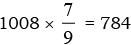QUESTION: 5

Directions: Study the table to answer the given questions:
Number of cars sold (in thousand) by 5 showrooms during 5 monthsQ. The no. of cars sold by showroom D in July is approximately what percent more than the number of cars sold by showroom A is March?

Solution: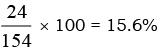QUESTION: 6

Directions: Study the table carefully and answer the given questions.
Number of Pages Printed by 6 Printers in 5 Different Weeks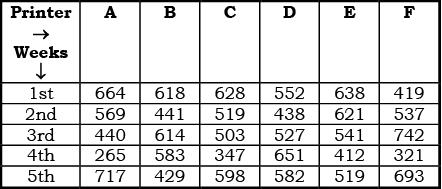Q. What is the respective ration between the number of pages printed by Printer – B in 2nd week and the number of pages printed by Printer – F in 5th week?

Solution:

441 : 693
7 : 11

QUESTION: 7

Directions: Study the table carefully and answer the given questions.
Number of Pages Printed by 6 Printers in 5 Different WeeksQ. What is the approx average number of pages printed by all the given printers in 4th week?

Solution: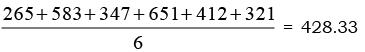≈ 425

QUESTION: 8

Directions: Study the table carefully and answer the given questions.
Number of Pages Printed by 6 Printers in 5 Different WeeksQ. Which of the following printer printed maximum number of pages in all the given weeks together?

Solution:
QUESTION: 9

Directions: Study the table carefully and answer the given questions.
Number of Pages Printed by 6 Printers in 5 Different WeeksQ. Number of pages printed by Printer – A in 3rd week is what percent of the total number of pages printed by Printer – D in all the given weeks?

Solution: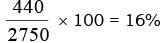QUESTION: 10

Directions: Study the table carefully and answer the given questions.
Number of Pages Printed by 6 Printers in 5 Different WeeksQ. What is the difference between the total number of pages printed by Printed – E in 1st, 2nd and 4th week together and total number of pages printed by Printer – C in all the given weeks together?

Solution:

Printer – E → I + II + IV Þ 638 + 621 + 421 = 1671
Printer – C + all ⇒ 628 + 519 + 503 + 347 + 598 = 2595
2595 – 1671 = 924

QUESTION: 11

Directions: Study the following pie-chart and answer the questions given below.
Preferences of Students for Six Beverages A, B, C, D, E and F in Terms of Degrees of Angle in the Pie-chart (Value in Degree)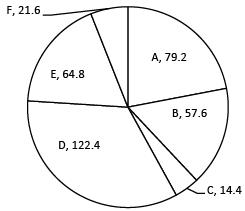Total No. of Students = 6800

Q. What is the difference between the total number of students who prefer beverage A and C together and the total number of students who prefer beverage D and F together?

Solution:

A + C = 79.2 + 14.4 = 93.6
D + F = 122.4 + 21.6 = 1440
Diff. = 144 – 93.6 = 50.4º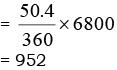QUESTION: 12

Directions: Study the following pie-chart and answer the questions given below.
Preferences of Students for Six Beverages A, B, C, D, E and F in Terms of Degrees of Angle in the Pie-chart (Value in Degree)Total No. of Students = 6800

Q. What is the ratio of the number of students who prefer beverage F to the number of students who prefer beverage A?

Solution:

21.6 : 79.2
3 : 11

QUESTION: 13

Directions: Study the following pie-chart and answer the questions given below.
Preferences of Students for Six Beverages A, B, C, D, E and F in Terms of Degrees of Angle in the Pie-chart (Value in Degree)Total No. of Students = 6800

Q. The number of students who prefer beverage E and F together is what percent of the total number of students?

Solution: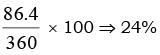QUESTION: 14

Directions: Study the following pie-chart and answer the questions given below.
Preferences of Students for Six Beverages A, B, C, D, E and F in Terms of Degrees of Angle in the Pie-chart (Value in Degree)Total No. of Students = 6800

Q. The number of students who prefer beverage C is approximately what percent of the number of students who prefer beverage D?

Solution: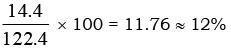QUESTION: 15

Directions: Study the following pie-chart and answer the questions given below.
Preferences of Students for Six Beverages A, B, C, D, E and F in Terms of Degrees of Angle in the Pie-chart (Value in Degree)Total No. of Students = 6800

Q. How many students prefer beverage B and E together?

Solution:

B + E = 57.6 + 64.8
= 122.4 s
Total number = (122.4/360) x 6800
= 2312